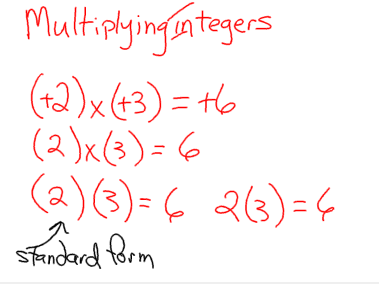## Thursday, March 10, 2011

### Roemer's Great book of integers

Chapter 1:
Integers

- number line
- Integer chipsNegative

PositiveZero Pair = 0

When subtracting something that isn't there, use a zero pair!(+4) + (-4)
= 0
have 4, owe 4

Training Wheels(+4) + (-4)
+4 - 4

4 - 4 <-- Standard Form.+10 - (-4)(-3) - (2) = -1

Questions:

(-3) - (-7) = +4Chapter 2:Examples:
(+2) x (+3) = 6
(+2) x (-3) = -6

(-2) x (+3) = 6
(-2) x (-3) = 6Chapter 3:
Dividing Integers;

Partitive division is when you are given a number of group and you are asked to find out how many things can be in each group.Quotative Division: